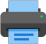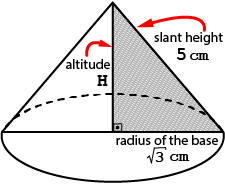Lista de exercícios do ensino médio para impressãoSelect all.
print selected exercises
What is the vertical height (altitude) of the right cone whose radius of the circular base is equal to $\;\sqrt{3}\,$ centimeters and the slant height is 5 centimeters ?

Thinking:

# The slant height of the cone is the distance from the apex to a point in the perimeter of the base. If the cone is a right cone, the length of all the slant heights are the same.Solution:
$\,\left.\begin{array}{rcr} \mbox{slant height }\phantom{XXXX}\;\,\rightarrow\, & \;S_h\mbox{ = 5 cm }\; \\ \,\mbox{radius of the base}\phantom{XX} \rightarrow\, & R\,=\,\sqrt{3}\\ \mbox{Pythagorean theorem}\, \rightarrow\, & (S_h)^{\large 2}\,=\,H^{\large 2}\,+\,R^{\large 2}\; \\ \end{array} \right\}\;\Rightarrow\;$
$\;\Rightarrow\;5^{\large 2}\,=\,H^{\large 2}\,+\,(\sqrt{3})^{\large 2}\;\Leftrightarrow\;H\,=\,\sqrt{22} \mbox{ cm}$
the vertical height of the cone is $\,H\,=\,\sqrt{22}\,$ cm
×
Other math tests:
right cone
altitude of the right cone
slant height